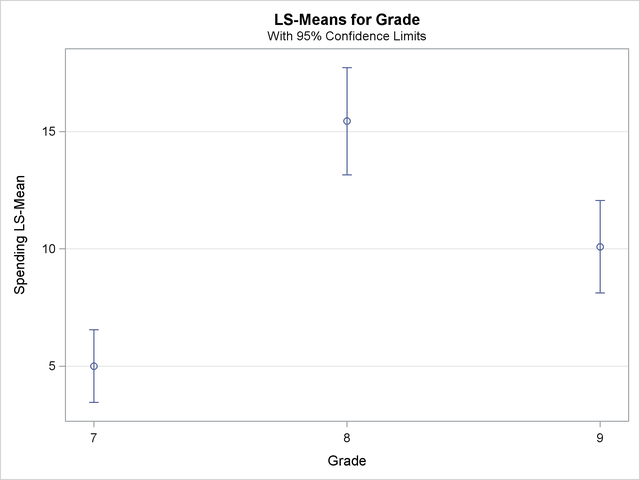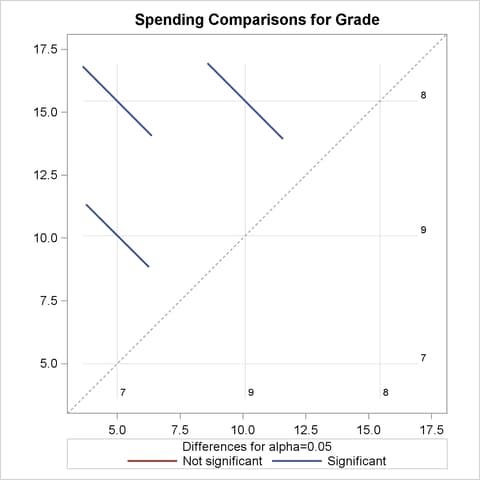Example 90.8 Compare Domain Statistics

This example is a continuation of Example 90.7 in which domain analyses for male and female students were performed. Suppose that you are now interested in estimating the gender domain means of weekly ice cream spending (that is, the average spending for males and females, respectively). You can use the SURVEYMEANS procedure to produce these domain statistics by using the following statements:

```proc surveymeans data=IceCreamDataDomain total=StudentTotals;
var spending;
domain Gender;
weight Weight;
run;
```

Output 90.8.1 shows the estimated spending among male and female students.

Output 90.8.1 Estimated Domain Means
The SURVEYMEANS Procedure

Domain Analysis: Gender
Gender Variable N Mean Std Error of Mean 95% CL for Mean
F Spending 19 9.376111 1.077927 7.19202418 11.5601988
M Spending 21 8.923052 1.003423 6.88992385 10.9561807

You can also use PROC SURVEYREG  to estimate these domain means. The benefit of this alternative approach is that PROC SURVEYREG  provides more tools for additional analysis, such as domain means comparisons in a LSMEANS statement.

Suppose that you want to test whether there is a significant difference for the ice cream spending between male and female students. You can use the following statements to perform the test:

```title1 'Ice Cream Spending Analysis';
title2 'Compare Domain Statistics';
class Gender;
model Spending = Gender / vadjust=none;
lsmeans Gender / diff;
weight Weight;
run;
```

The variable Gender is used as a model effect. The VADJUST=NONE option is used to produce variance estimates for domain means that are identical to those produced by PROC SURVEYMEANS. The LSMEANS statement requests that PROC SURVEYREG  estimate the average spending in each gender group. The DIFF option requests that the procedure compute the difference among domain means.

Output 90.8.2 displays the estimated weekly spending on ice cream among male and female students, respectively, and their standard errors. Female students spend \$9.38 per week on average, and male students spend \$8.92 per week on average. These domain means, including their standard errors, are identical to those in Output 90.8.1 which are produced by PROC SURVEYMEANS.

Output 90.8.2 Domain Means between Gender
 Ice Cream Spending Analysis Compare Domain Statistics

The SURVEYREG Procedure

Regression Analysis for Dependent Variable Spending

Gender Least Squares Means
Gender Estimate Standard Error DF t Value Pr > |t|
F 9.3761 1.0779 37 8.70 <.0001
M 8.9231 1.0034 37 8.89 <.0001

Output 90.8.3 shows the estimated difference for weekly ice scream spending between the two gender groups. The female students spend \$0.45 more than male students on average, and the difference is not statistically significant based on the t test.

Output 90.8.3 Domain Means Comparison
Differences of Gender Least Squares Means
Gender _Gender Estimate Standard Error DF t Value Pr > |t|
F M 0.4531 1.7828 37 0.25 0.8008

If you want to investigate whether there is any significant difference in ice cream spending among grades, you can use the following similar statements to compare:

```title1 'Ice Cream Spending Analysis';
title2 'Compare Domain Statistics';
ods graphics on;
lsmeans Grade / diff plots=(diff meanplot(cl));
weight Weight;
run;
ods graphics off;
```

The Grade is specified in the CLASS statement to be used as an effect in the MODEL statement. The DIFF option in the LSMEANS statement requests that the procedure compute the difference among the domain means for the effect Grade. The ODS GRAPHICS statement enables ODS to create graphics. The PLOTS=(DIFF MEANPLOT(CL)) option requests two graphics: the domain means plot "MeanPlot" and their pairwise difference plot "DiffPlot". The CL suboption requests the "MeanPlot" to display confidence. For information about ODS Graphics, see Chapter 21, Statistical Graphics Using ODS.

Output 90.8.4 shows the estimated weekly spending on ice cream for students within each grade. Students in Grade 7 spend the least, only \$5.00 per week. Students in Grade 8 spend the most, \$15.44 per week. Students in Grade 9 spend a little less at \$10.09 per week.

Output 90.8.4 Domain Means among Grades
 Ice Cream Spending Analysis Compare Domain Statistics

The SURVEYREG Procedure

Regression Analysis for Dependent Variable Spending

Grade Estimate Standard Error DF t Value Pr > |t|
7 5.0000 0.7636 37 6.55 <.0001
8 15.4444 1.1268 37 13.71 <.0001
9 10.0909 0.9719 37 10.38 <.0001

Output 90.8.5 plots the weekly spending results that are shown in Output 90.8.4.

Output 90.8.5 Plot of Means of Ice Cream Spending within GradesOutput 90.8.6 displays pairwise comparisons for weekly ice scream spending among grades. All the differences are significant based on t tests.

Output 90.8.6 Domain Means Comparison
Differences of Grade Least Squares Means